The Python Toolbox for Neurophysiological Signal Processing.

## Project descriptionThe Python Toolbox for Neurophysiological Signal Processing

NeuroKit2 is a user-friendly package providing easy access to advanced biosignal processing routines. Researchers and clinicians without extensive knowledge of programming or biomedical signal processing can analyze physiological data with only two lines of code.

## Quick Example

import neurokit2 as nk

data = nk.data("bio_eventrelated_100hz")

# Preprocess the data (filter, find peaks, etc.)
processed_data, info = nk.bio_process(ecg=data["ECG"], rsp=data["RSP"], eda=data["EDA"], sampling_rate=100)

# Compute relevant features
results = nk.bio_analyze(processed_data, sampling_rate=100)

And boom 💥 your analysis is done 😎

pip install neurokit2
conda install -c conda-forge neurokit2

If you’re not sure what to do, read our installation guide.

## ContributingNeuroKit2 is the most welcoming project with a large community of contributors with all levels of programming expertise. But the package is still far from being perfect! Thus, if you have some ideas for improvement, new features, or just want to learn Python and do something useful at the same time, do not hesitate and check out the following guide:

Also, if you have developed new signal processing methods or algorithms and you want to increase their usage, popularity, and citations, get in touch with us to eventually add them to NeuroKit. A great opportunity for the users as well as the original developers!

You have spotted a mistake? An error in a formula or code? OR there is just a step that seems strange and you don’t understand? Please let us know! We are human beings, and we’ll appreciate any inquiry.

## DocumentationClick on the links above and check out our tutorials:

### Examples

Don’t know which tutorial is suited for your case? Follow this flowchart: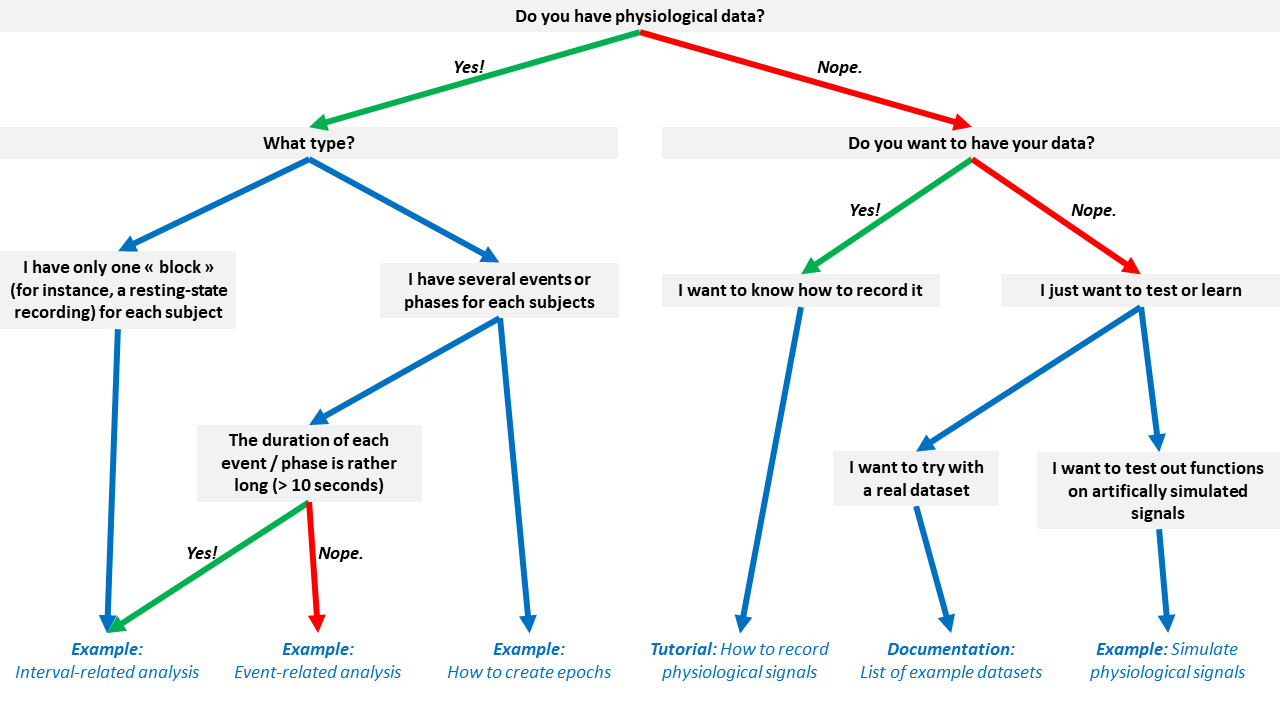## CitationThe NeuroKit2 paper can be found here 🎉 Additionally, you can get the reference directly from Python by running:

nk.cite()
You can cite NeuroKit2 as follows:

- Makowski, D., Pham, T., Lau, Z. J., Brammer, J. C., Lespinasse, F., Pham, H.,
Schölzel, C., & Chen, S. A. (2021). NeuroKit2: A Python toolbox for neurophysiological signal processing.
Behavior Research Methods, 53(4), 1689–1696. https://doi.org/10.3758/s13428-020-01516-y

Full bibtex reference:

@article{Makowski2021neurokit,
author = {Dominique Makowski and Tam Pham and Zen J. Lau and Jan C. Brammer and Fran{\c{c}}ois Lespinasse and Hung Pham and Christopher Schölzel and S. H. Annabel Chen},
title = {{NeuroKit}2: A Python toolbox for neurophysiological signal processing},
journal = {Behavior Research Methods},
volume = {53},
number = {4},
pages = {1689--1696},
publisher = {Springer Science and Business Media {LLC}},
doi = {10.3758/s13428-020-01516-y},
url = {https://doi.org/10.3758%2Fs13428-020-01516-y},
year = 2021,
month = {feb}
}

Let us know if you used NeuroKit2 in a publication! Open a new discussion (select the NK in publications category) and link the paper. The community would be happy to know about how you used it and learn about your research. We could also feature it once we have a section on the website for papers that used the software.

## Physiological Data Preprocessing

### Simulate physiological signals

You can easily simulate artificial ECG (also 12-Lead multichannel ECGs), PPG, RSP, EDA, and EMG signals to test your scripts and algorithms.

import numpy as np
import pandas as pd
import neurokit2 as nk

# Generate synthetic signals
ecg = nk.ecg_simulate(duration=10, heart_rate=70)
ppg = nk.ppg_simulate(duration=10, heart_rate=70)
rsp = nk.rsp_simulate(duration=10, respiratory_rate=15)
eda = nk.eda_simulate(duration=10, scr_number=3)
emg = nk.emg_simulate(duration=10, burst_number=2)

# Visualise biosignals
data = pd.DataFrame({"ECG": ecg,
"PPG": ppg,
"RSP": rsp,
"EDA": eda,
"EMG": emg})
nk.signal_plot(data, subplots=True)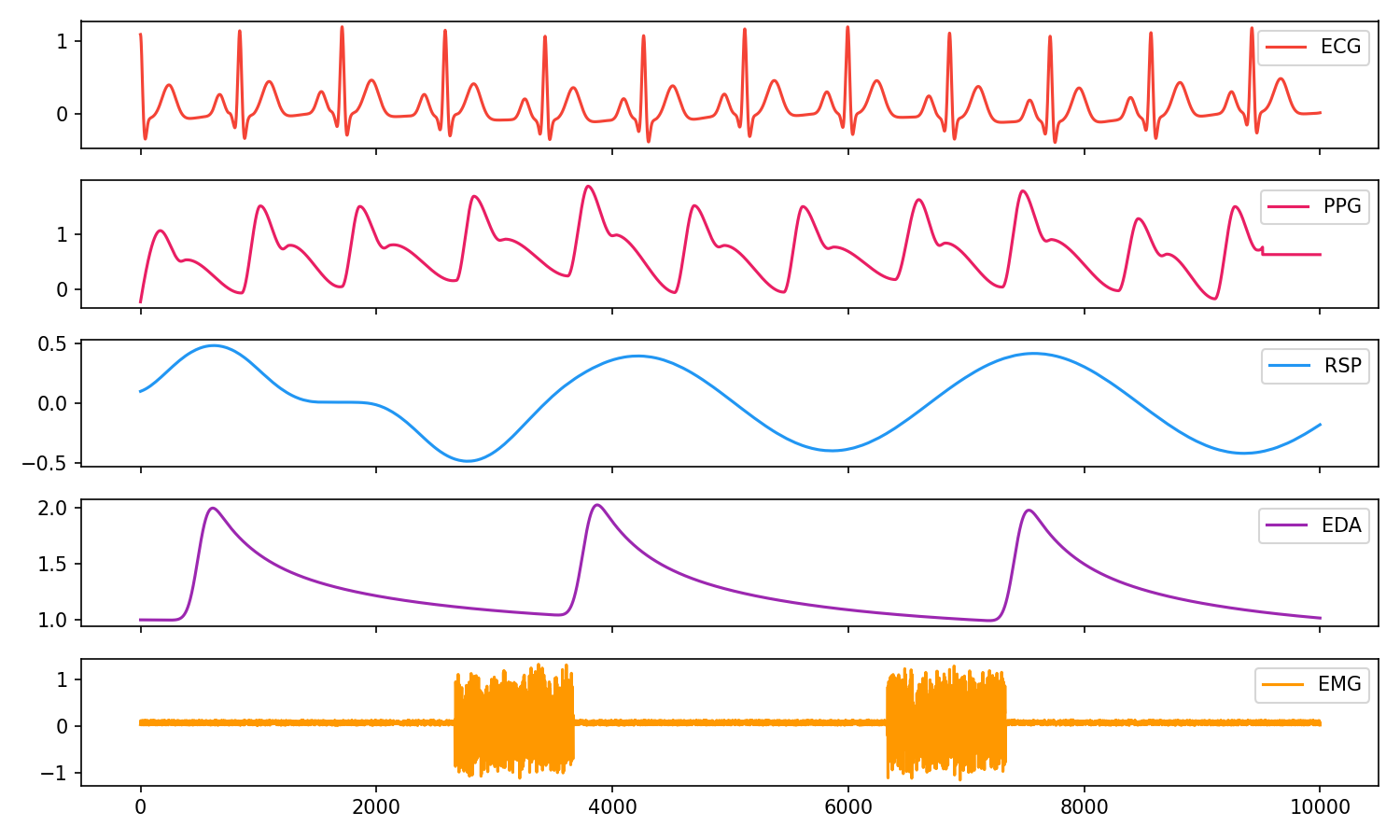### Electrodermal Activity (EDA/GSR)

# Generate 10 seconds of EDA signal (recorded at 250 samples / second) with 2 SCR peaks
eda = nk.eda_simulate(duration=10, sampling_rate=250, scr_number=2, drift=0.01)

# Process it
signals, info = nk.eda_process(eda, sampling_rate=250)

# Visualise the processing
nk.eda_plot(signals, info)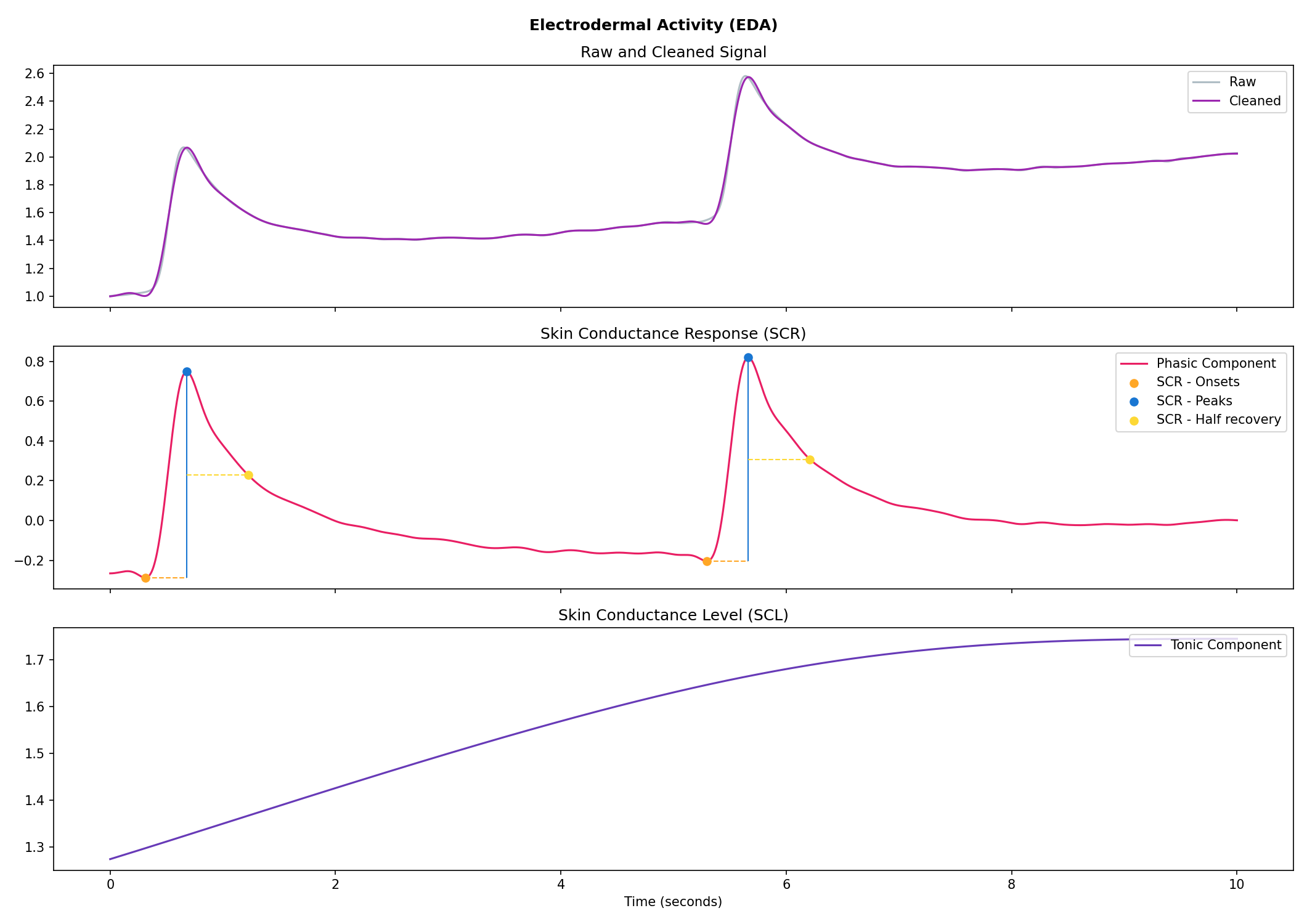### Cardiac activity (ECG)

# Generate 15 seconds of ECG signal (recorded at 250 samples/second)
ecg = nk.ecg_simulate(duration=15, sampling_rate=250, heart_rate=70)

# Process it
signals, info = nk.ecg_process(ecg, sampling_rate=250)

# Visualise the processing
nk.ecg_plot(signals, info)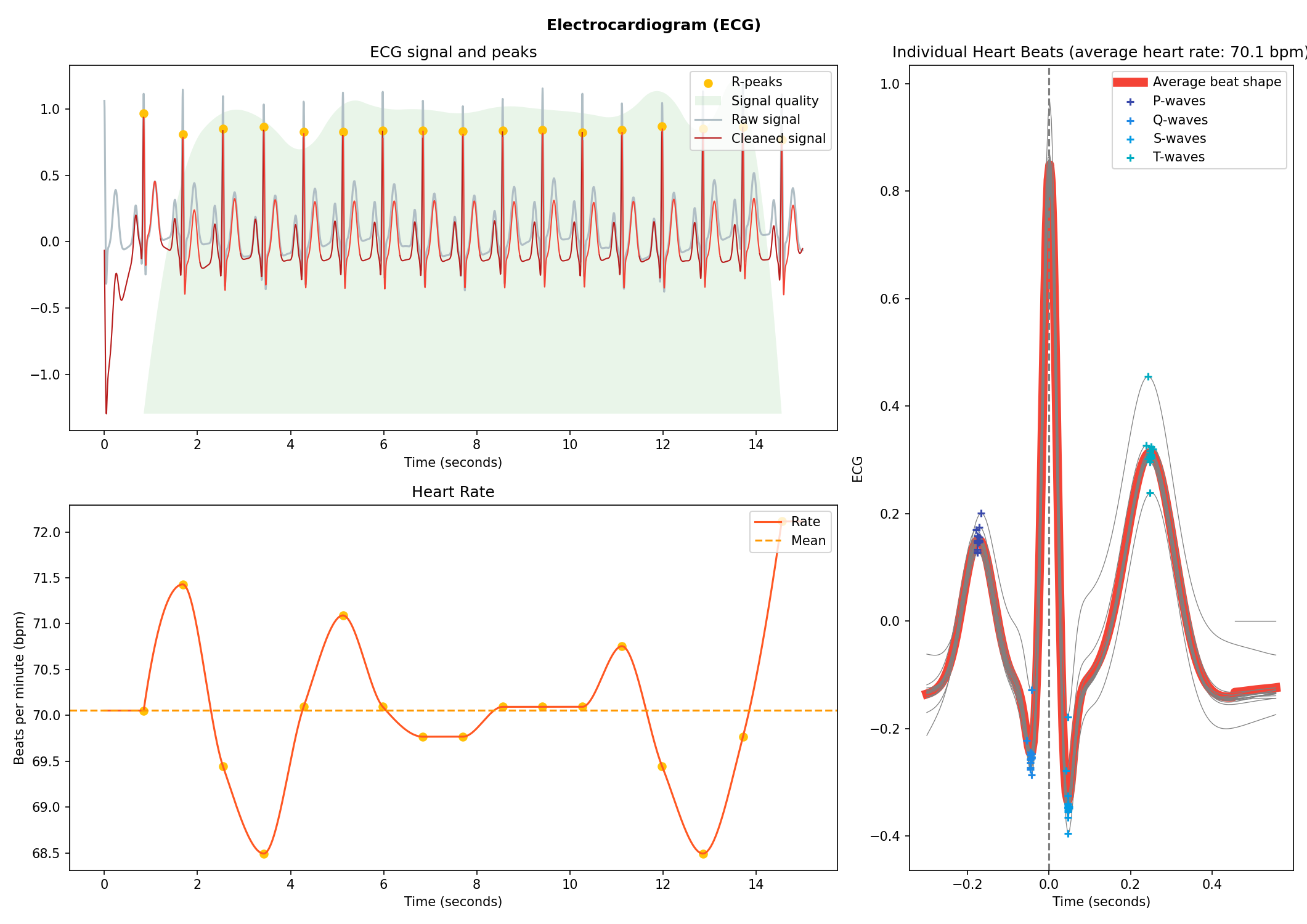### Respiration (RSP)

# Generate one minute of respiratory (RSP) signal (recorded at 250 samples / second)
rsp = nk.rsp_simulate(duration=60, sampling_rate=250, respiratory_rate=15)

# Process it
signals, info = nk.rsp_process(rsp, sampling_rate=250)

# Visualise the processing
nk.rsp_plot(signals, info)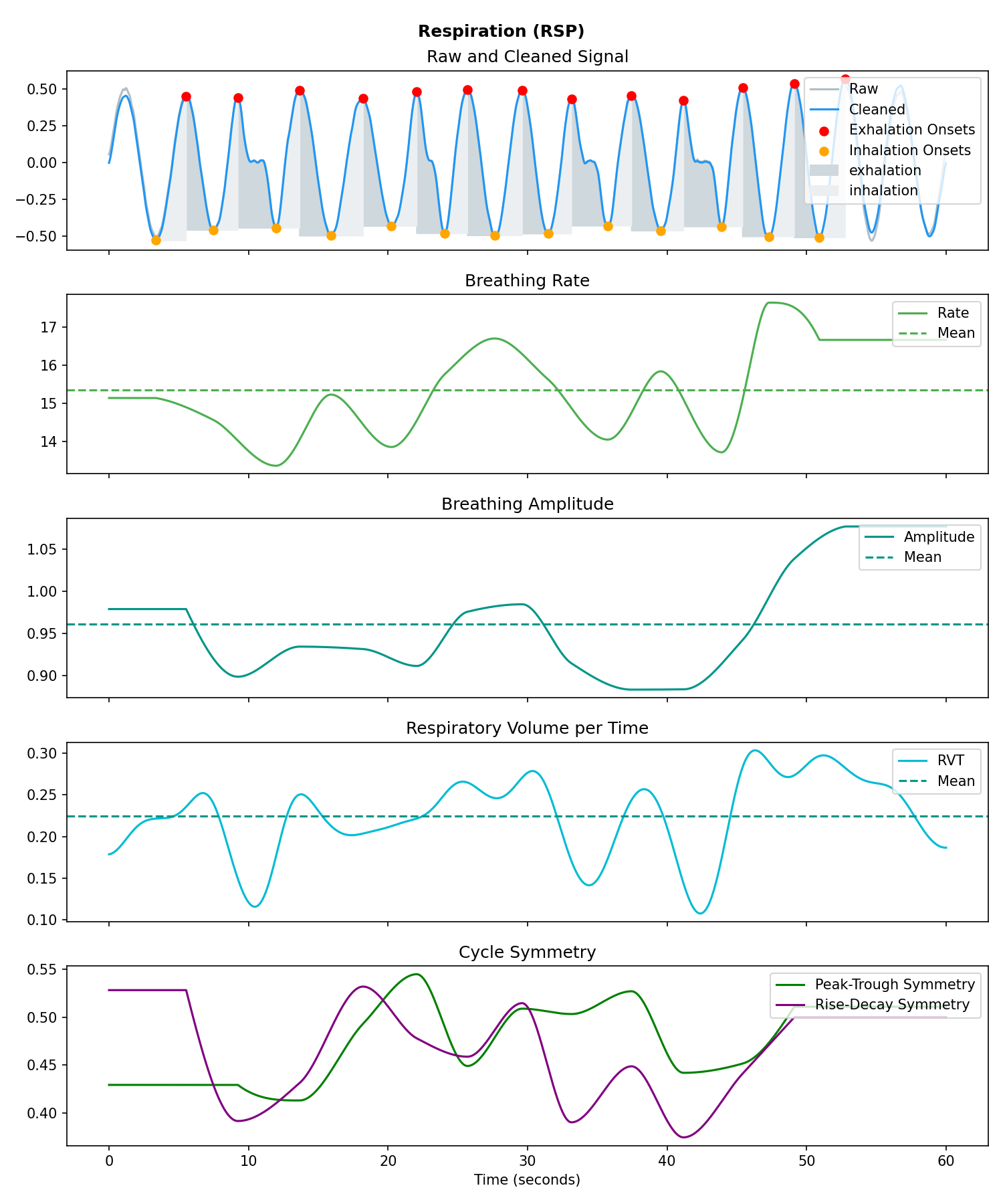### Photoplethysmography (PPG/BVP)

# Generate 15 seconds of PPG signal (recorded at 250 samples/second)
ppg = nk.ppg_simulate(duration=15, sampling_rate=250, heart_rate=70)

# Process it
signals, info = nk.ppg_process(ppg, sampling_rate=250)

# Visualize the processing
nk.ppg_plot(signals, info)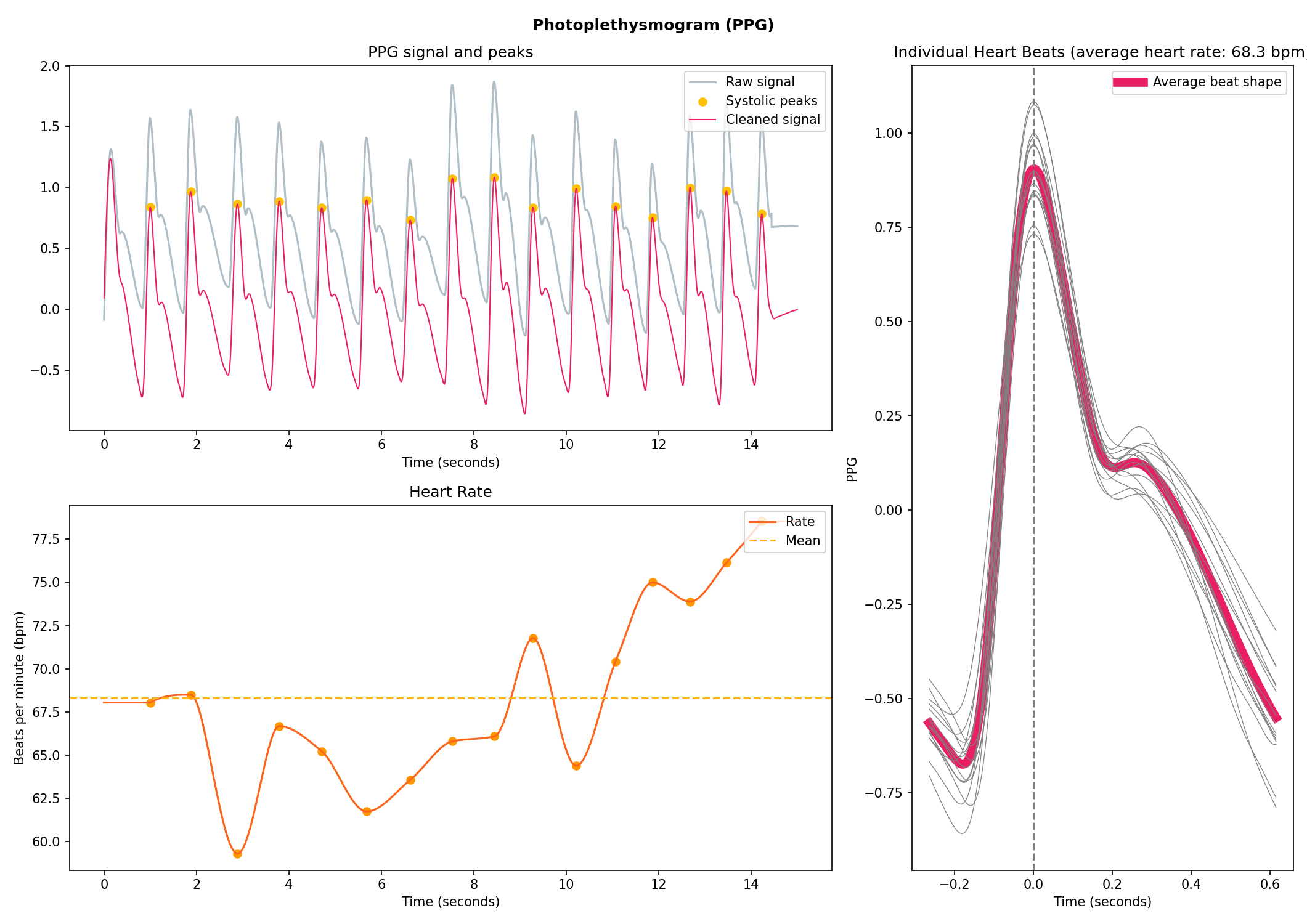### Electromyography (EMG)

# Generate 10 seconds of EMG signal (recorded at 250 samples/second)
emg = nk.emg_simulate(duration=10, sampling_rate=250, burst_number=3)

# Process it
signals, info = nk.emg_process(emg, sampling_rate=250)

# Visualise the processing
nk.emg_plot(signals, info)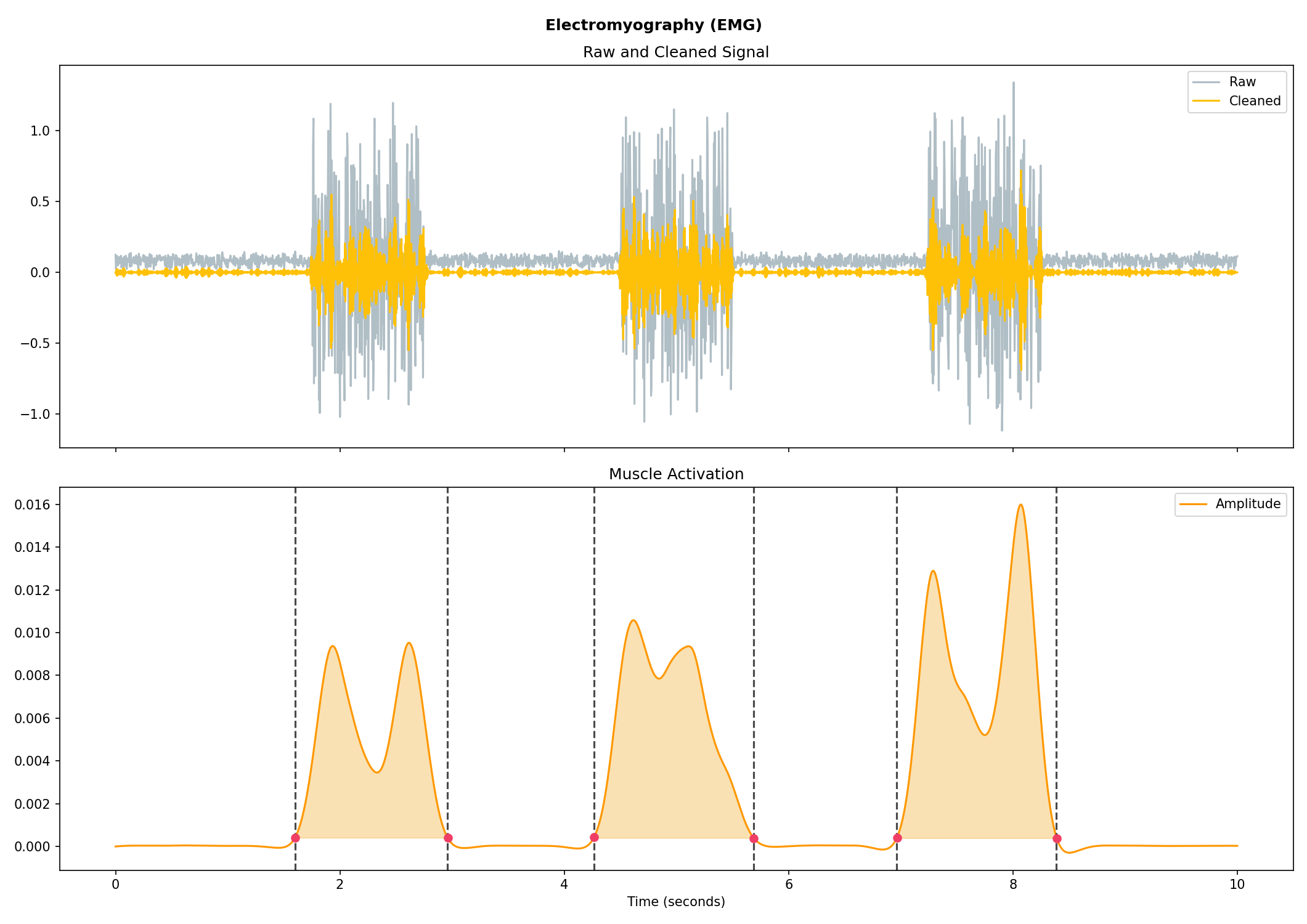### Electrooculography (EOG)

# Import EOG data
eog_signal = nk.data("eog_100hz")

# Process it
signals, info = nk.eog_process(eog_signal, sampling_rate=100)

# Plot
nk.eog_plot(signals, info)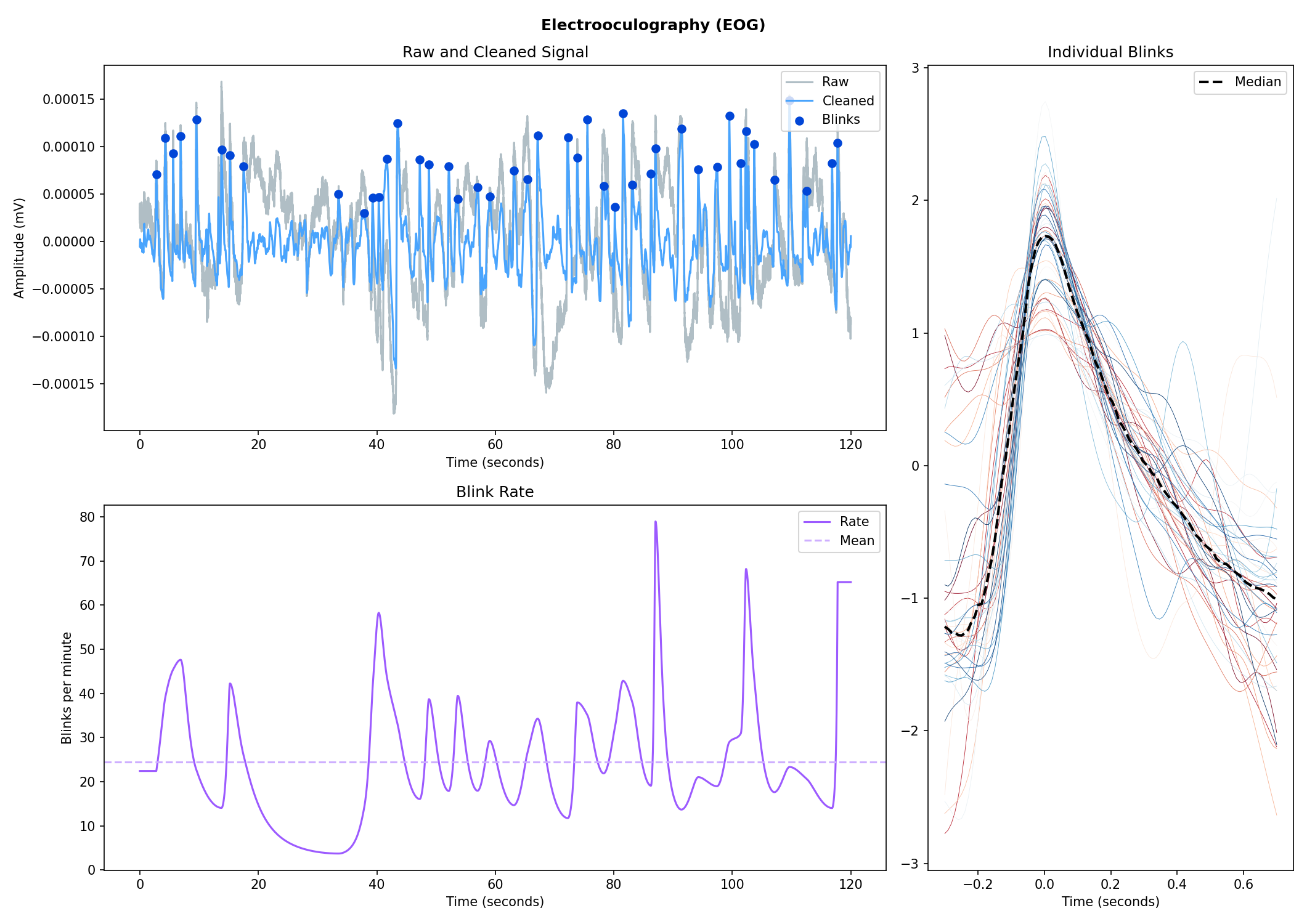### Electrogastrography (EGG)

Consider helping us develop it!

## Physiological Data Analysis

The analysis of physiological data usually comes in two types, event-related or interval-related.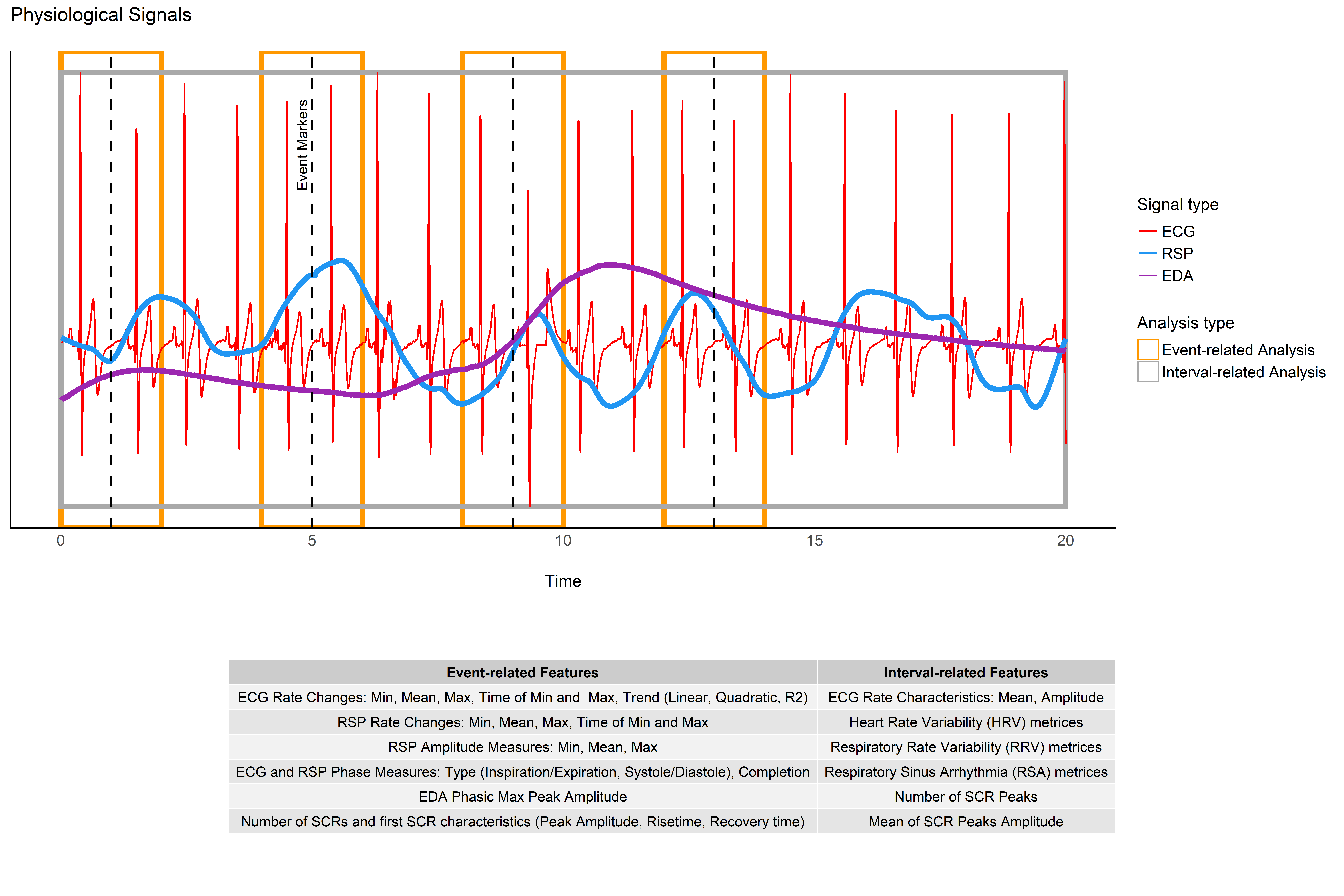## Heart Rate Variability (HRV)Check-out our Heart Rate Variability in Psychology: A Review of HRV Indices and an Analysis Tutorial paper for:

• a comprehensive review of the most up-to-date HRV indices

• a discussion of their significance in psychological research and practices

• a step-by-step guide for HRV analysis using NeuroKit2

You can cite the paper as follows:

- Pham, T., Lau, Z. J., Chen, S. H. A., & Makowski, D. (2021).
Heart Rate Variability in Psychology: A Review of HRV Indices and an Analysis Tutorial.
Sensors, 21(12), 3998. https://doi:10.3390/s21123998
• Compute HRV indices using Python

• Time domain: RMSSD, MeanNN, SDNN, SDSD, CVNN, etc.

• Frequency domain: Spectral power density in various frequency bands (Ultra low/ULF, Very low/VLF, Low/LF, High/HF, Very high/VHF), Ratio of LF to HF power, Normalized LF (LFn) and HF (HFn), Log transformed HF (LnHF).

• Nonlinear domain: Spread of RR intervals (SD1, SD2, ratio between SD2 to SD1), Cardiac Sympathetic Index (CSI), Cardial Vagal Index (CVI), Modified CSI, Sample Entropy (SampEn).

# Download data
data = nk.data("bio_resting_8min_100hz")

# Find peaks
peaks, info = nk.ecg_peaks(data["ECG"], sampling_rate=100)

# Compute HRV indices
nk.hrv(peaks, sampling_rate=100, show=True)
>>>    HRV_RMSSD  HRV_MeanNN   HRV_SDNN  ...   HRV_CVI  HRV_CSI_Modified  HRV_SampEn
>>> 0  69.697983  696.395349  62.135891  ...  4.829101        592.095372    1.259931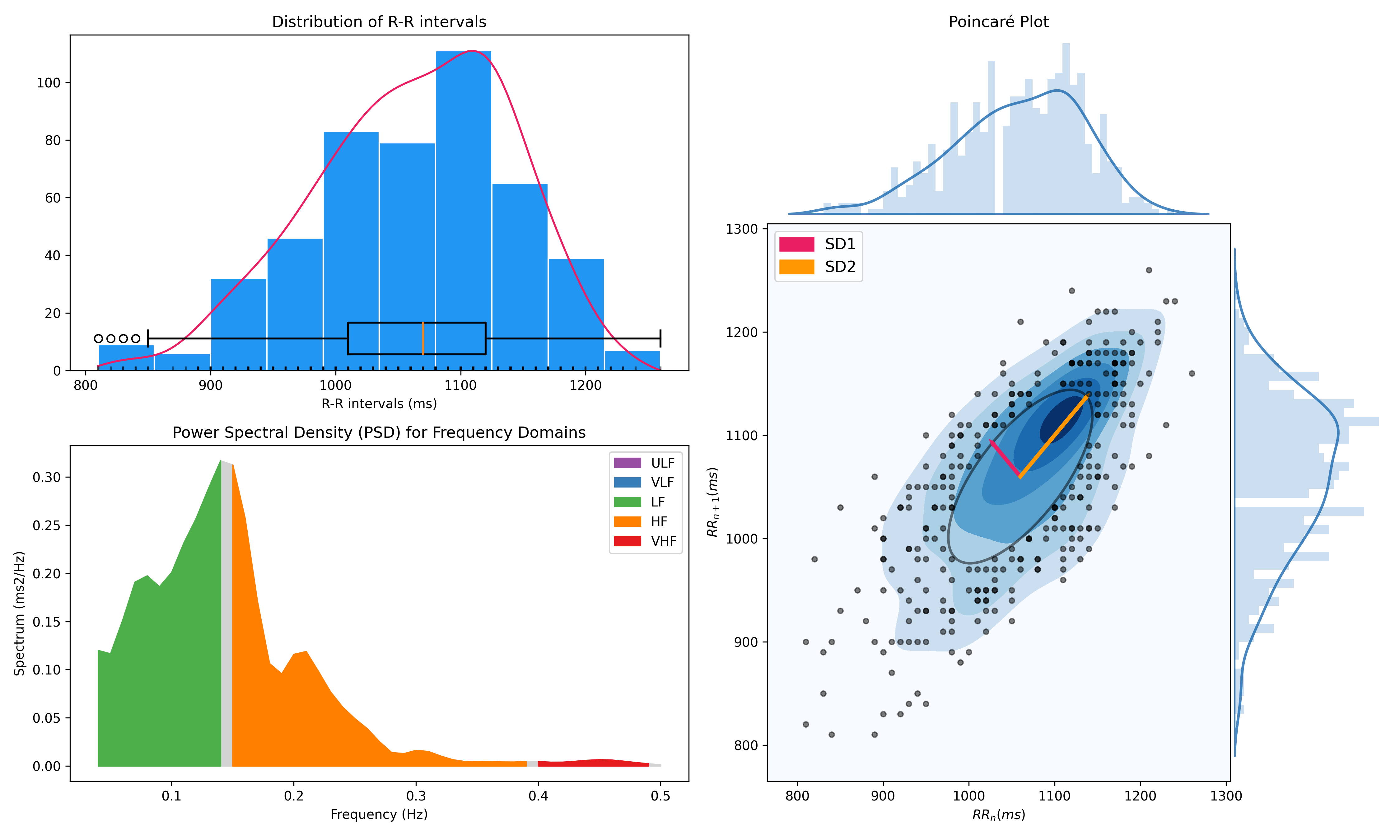## Miscellaneous

### ECG Delineation

• Delineate the QRS complex of an electrocardiac signal (ECG) including P-peaks, T-peaks, as well as their onsets and offsets.

# Download data
ecg_signal = nk.data(dataset="ecg_3000hz")

# Extract R-peaks locations
_, rpeaks = nk.ecg_peaks(ecg_signal, sampling_rate=3000)

# Delineate
signal, waves = nk.ecg_delineate(ecg_signal, rpeaks, sampling_rate=3000, method="dwt", show=True, show_type='all')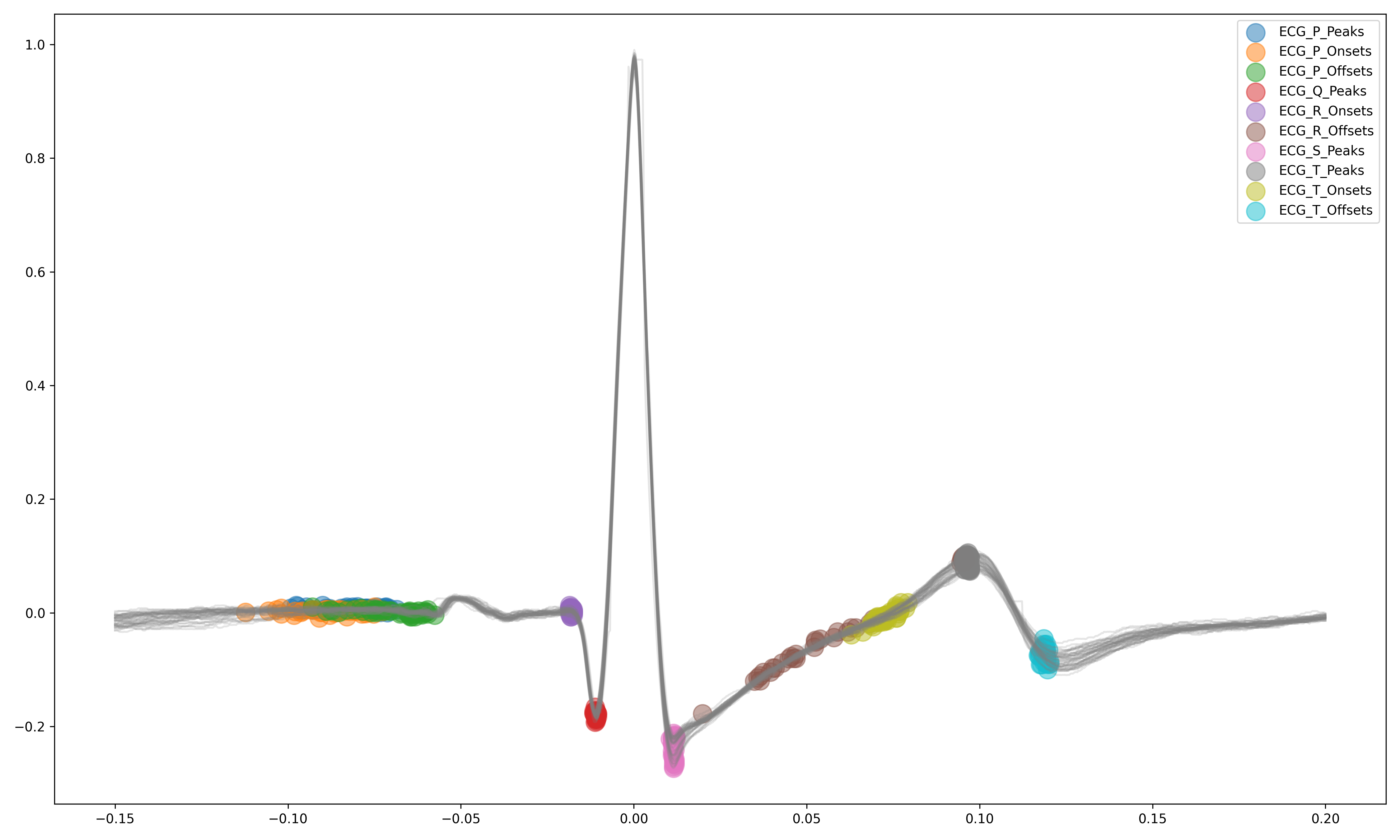### Signal Processing

• Signal processing functionalities

• Filtering: Using different methods.

• Detrending: Remove the baseline drift or trend.

• Distorting: Add noise and artifacts.

# Generate original signal
original = nk.signal_simulate(duration=6, frequency=1)

# Distort the signal (add noise, linear trend, artifacts, etc.)
distorted = nk.signal_distort(original,
noise_amplitude=0.1,
noise_frequency=[5, 10, 20],
powerline_amplitude=0.05,
artifacts_amplitude=0.3,
artifacts_number=3,
linear_drift=0.5)

# Clean (filter and detrend)
cleaned = nk.signal_detrend(distorted)
cleaned = nk.signal_filter(cleaned, lowcut=0.5, highcut=1.5)

# Compare the 3 signals
plot = nk.signal_plot([original, distorted, cleaned])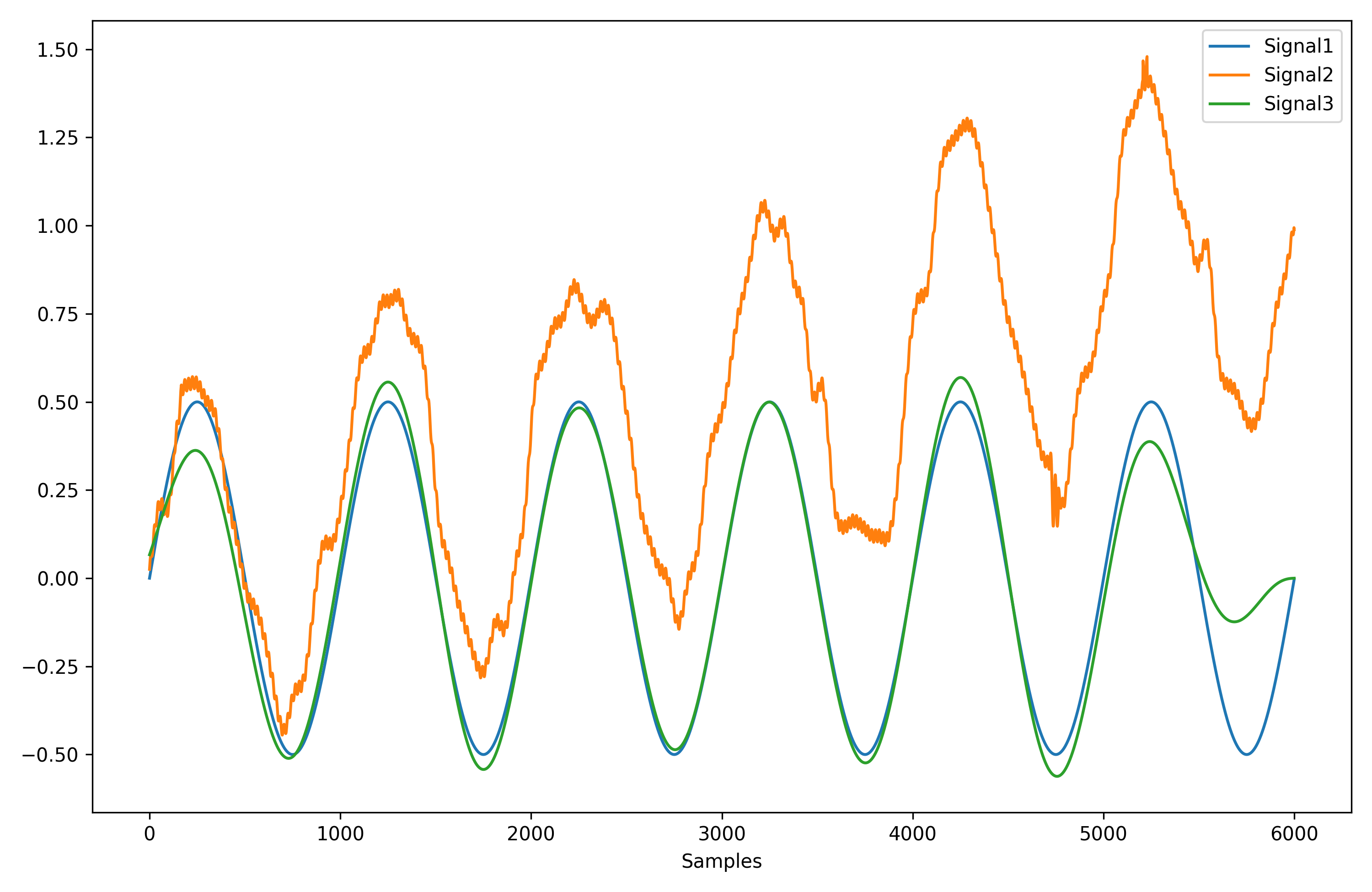### Complexity (Entropy, Fractal Dimensions, …)

• Optimize complexity parameters (delay tau, dimension m, tolerance r)

# Generate signal
signal = nk.signal_simulate(frequency=[1, 3], noise=0.01, sampling_rate=200)

# Find optimal time delay, embedding dimension, and r
parameters = nk.complexity_optimize(signal, show=True)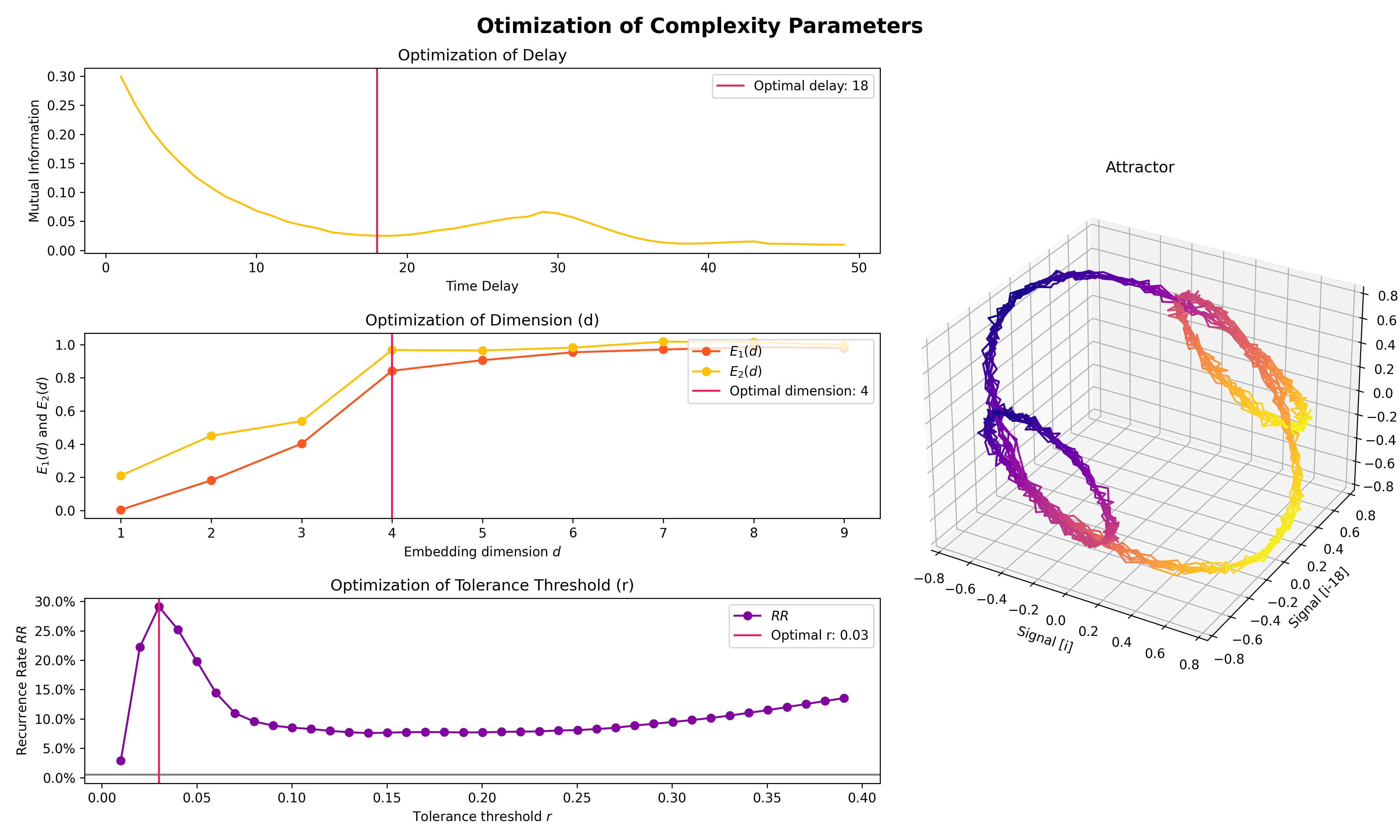• Compute complexity features

• Entropy: Sample Entropy (SampEn), Approximate Entropy (ApEn), Fuzzy Entropy (FuzzEn), Multiscale Entropy (MSE), Shannon Entropy (ShEn)

• Fractal dimensions: Correlation Dimension D2, …

• Detrended Fluctuation Analysis

nk.entropy_sample(signal)
nk.entropy_approximate(signal)

### Signal Decomposition

# Create complex signal
signal = nk.signal_simulate(duration=10, frequency=1)  # High freq
signal += 3 * nk.signal_simulate(duration=10, frequency=3)  # Higher freq
signal += 3 * np.linspace(0, 2, len(signal))  # Add baseline and linear trend
signal += 2 * nk.signal_simulate(duration=10, frequency=0.1, noise=0)  # Non-linear trend
signal += np.random.normal(0, 0.02, len(signal))  # Add noise

# Decompose signal using Empirical Mode Decomposition (EMD)
components = nk.signal_decompose(signal, method='emd')
nk.signal_plot(components)  # Visualize components

# Recompose merging correlated components
recomposed = nk.signal_recompose(components, threshold=0.99)
nk.signal_plot(recomposed)  # Visualize components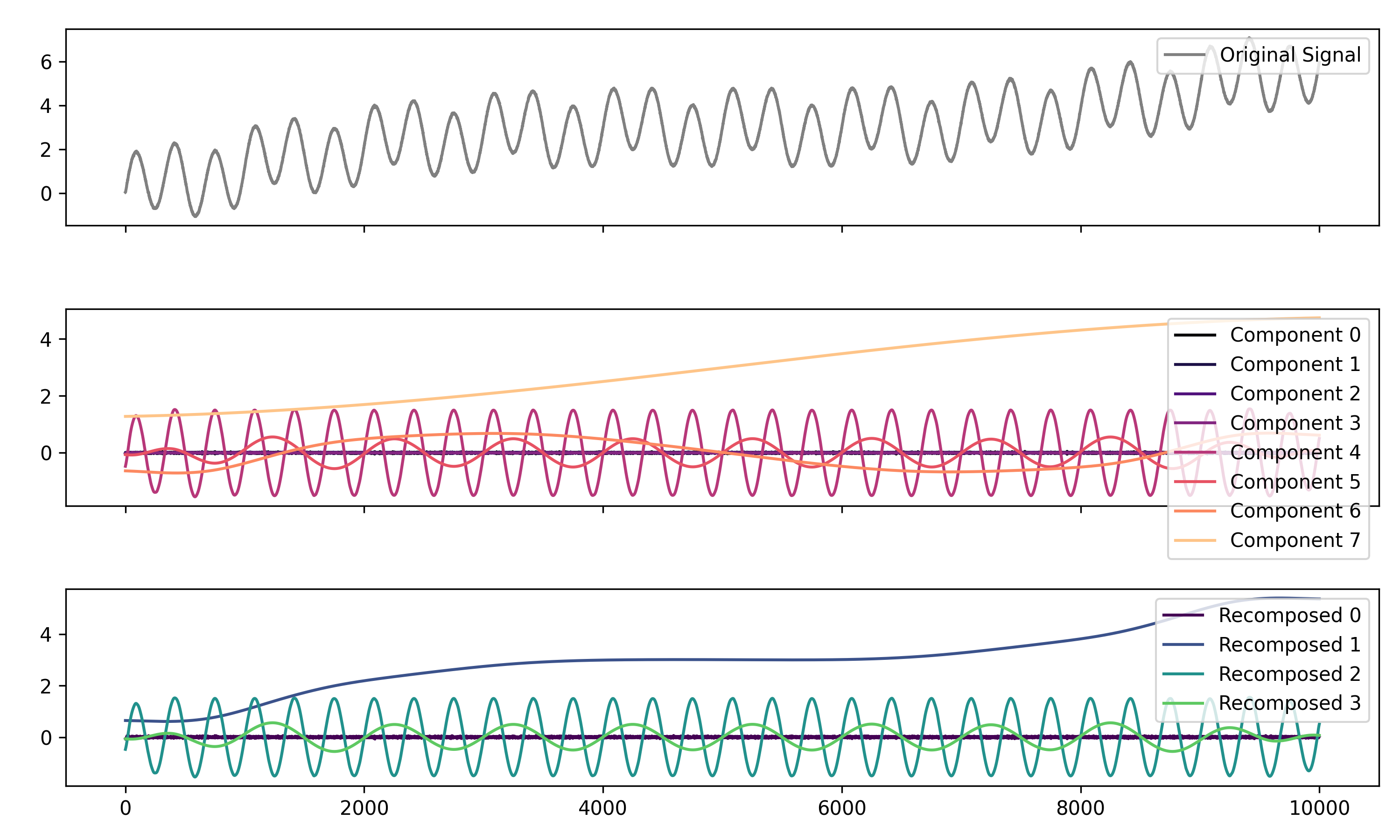### Signal Power Spectrum Density (PSD)

# Generate complex signal
signal = nk.signal_simulate(duration=20, frequency=[0.5, 5, 10, 15], amplitude=[2, 1.5, 0.5, 0.3], noise=0.025)

# Get the PSD using different methods
welch = nk.signal_psd(signal, method="welch", min_frequency=1, max_frequency=20, show=True)
multitaper = nk.signal_psd(signal, method="multitapers", max_frequency=20, show=True)
lomb = nk.signal_psd(signal, method="lomb", min_frequency=1, max_frequency=20, show=True)
burg = nk.signal_psd(signal, method="burg", min_frequency=1, max_frequency=20, order=10, show=True)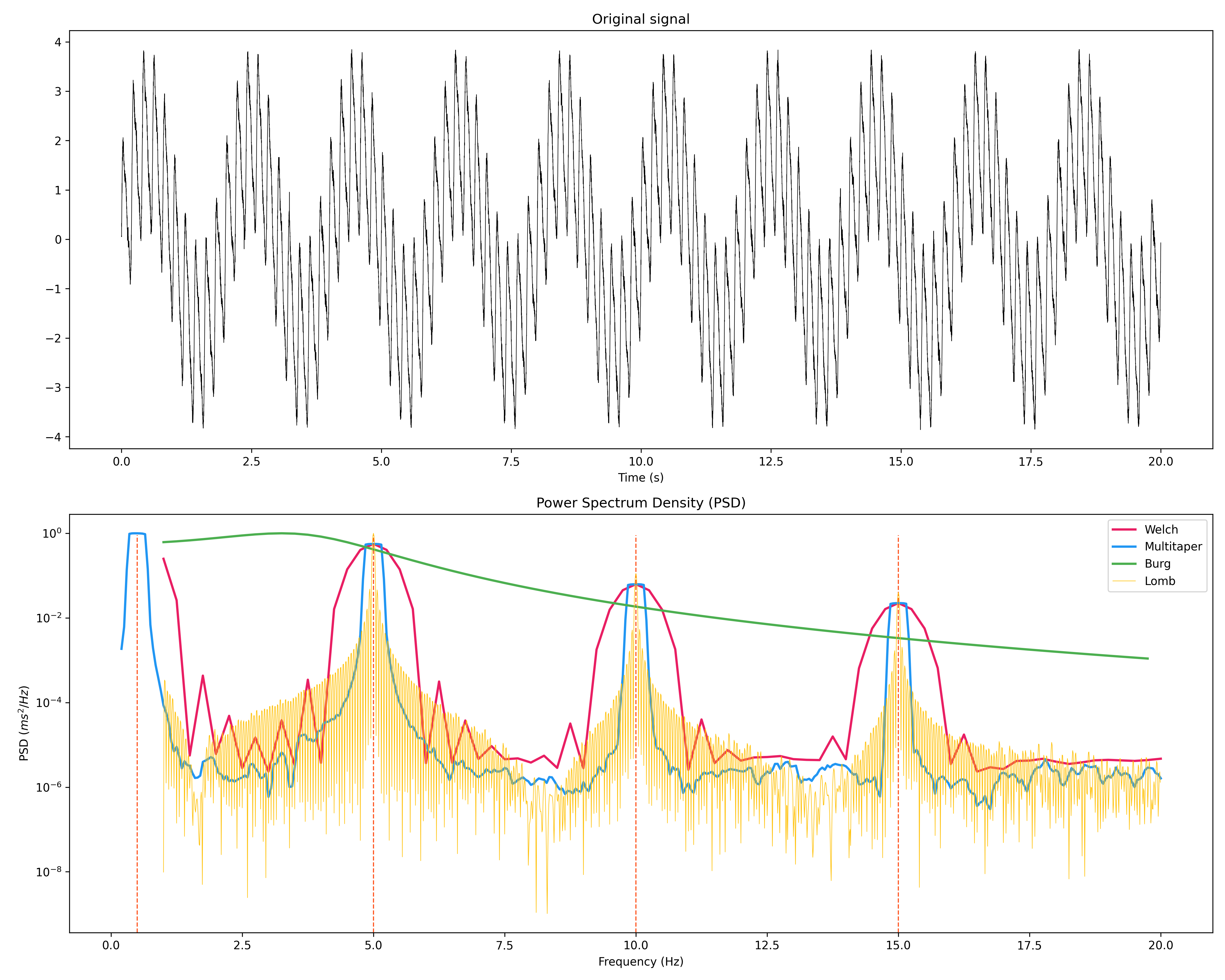### Statistics

• Highest Density Interval (HDI)

x = np.random.normal(loc=0, scale=1, size=100000)

ci_min, ci_max = nk.hdi(x, ci=0.95, show=True)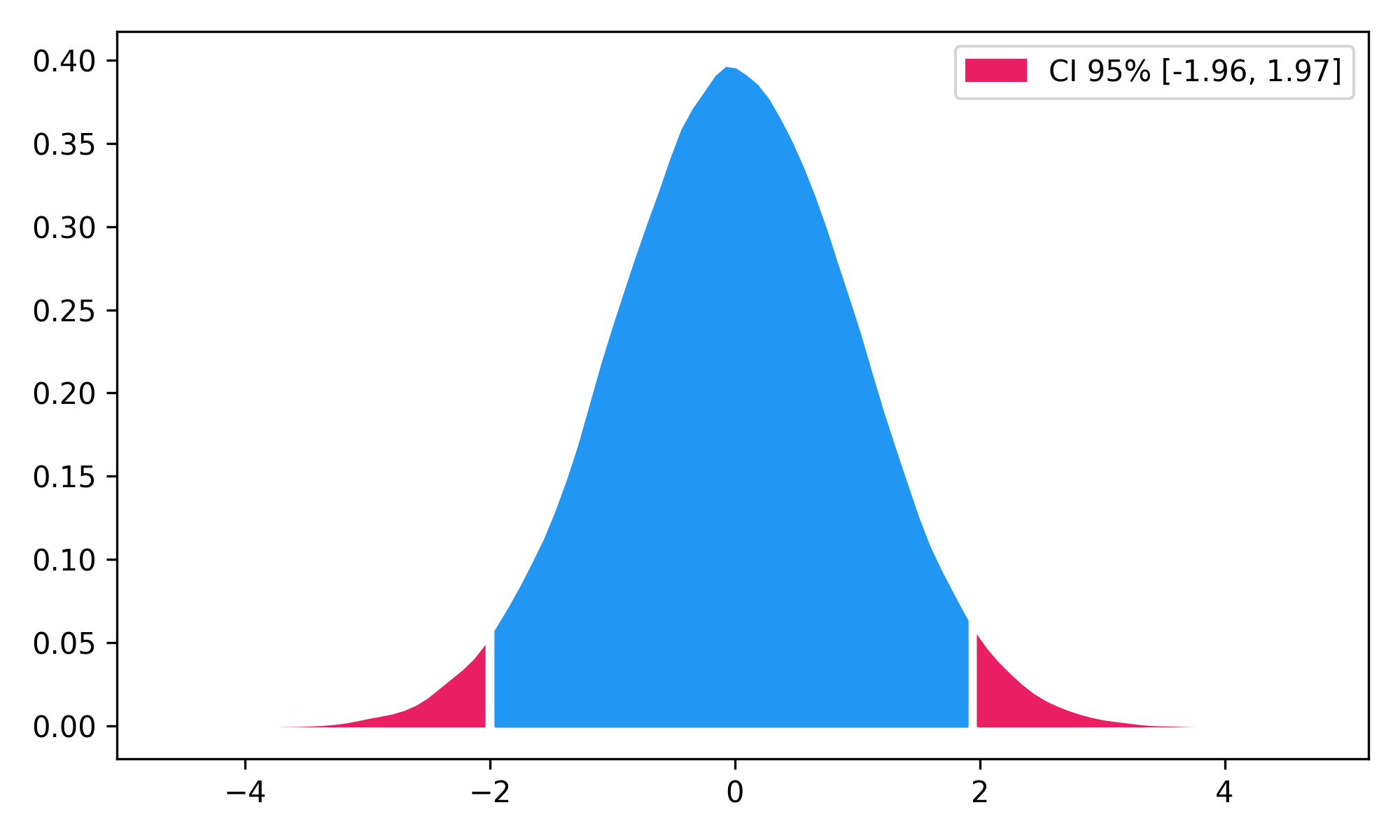## PopularityNeuroKit2 is one of the most welcoming packages for new contributors and users, as well as the fastest-growing package. So stop hesitating and hop on board 🤗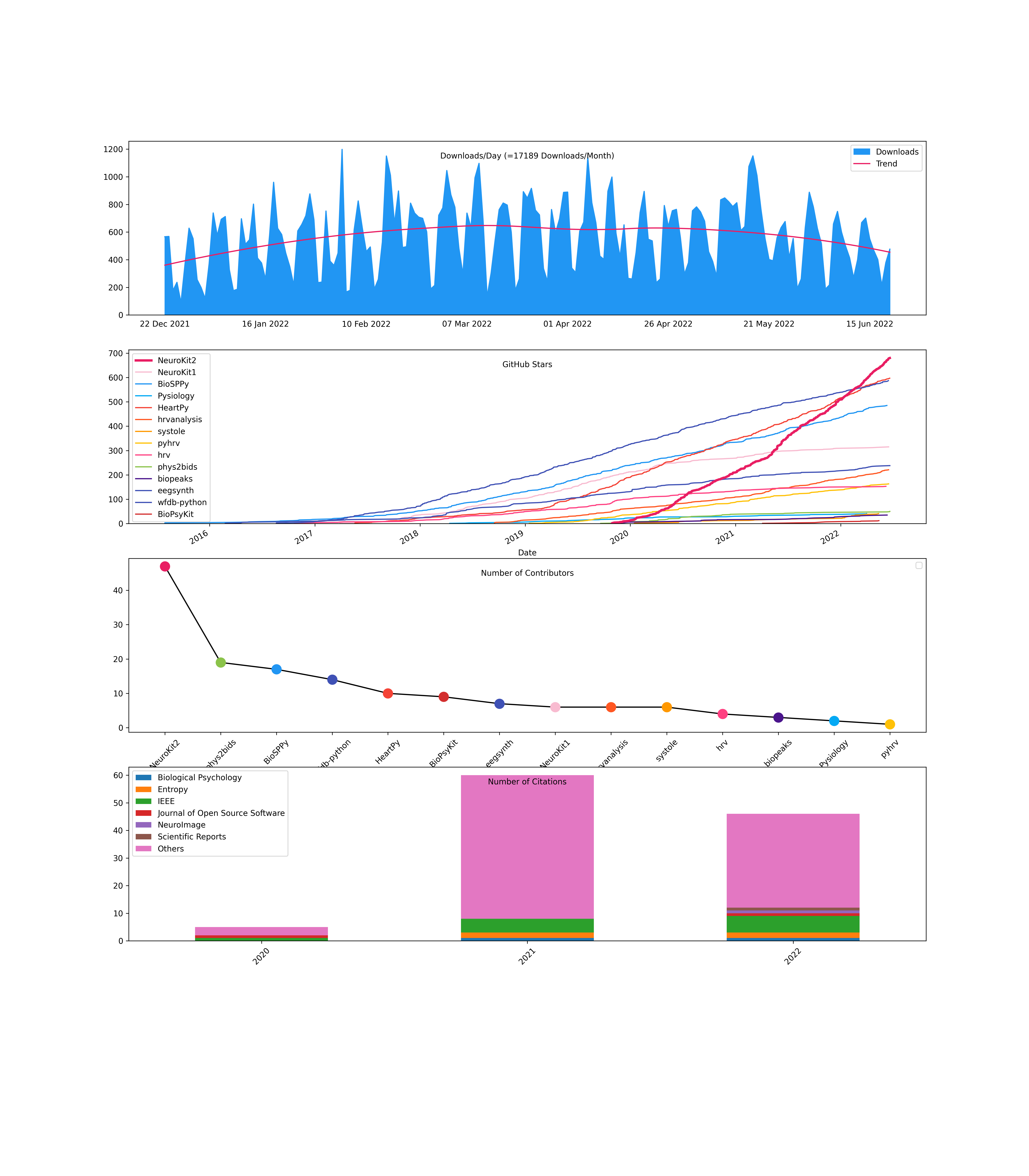## Used at## Disclaimer

The authors do not provide any warranty. If this software causes your keyboard to blow up, your brain to liquefy, your toilet to clog or a zombie plague to break loose, the authors CANNOT IN ANY WAY be held responsible.

## News

### 0.2.4

Fixes

• eda_sympathetic() has been reviewed: low-pass filter and resampling have been added to be in line with the original paper

• eda_findpeaks() using methods proposed in nabian2018 is reviewed and improved. Differentiation has been added before smoothing. Skin conductance response criteria have been revised based on the original paper.

### 0.2.1

New Features

• Allow for input with NaNs and extrapolation in signal_interpolate()

• Add argument method in find_outliers()

### 0.2.0

New Features

• Add new time-domain measures in hrv_time(): Prc20NN, Prc80NN, MinNN, and MaxNN

### 0.1.6

#### Breaking Changes

• Argument type changed to out in expspace()

#### New Features

• Add new time-domain measures in hrv_time(): Prc20NN, Prc80NN, MinNN, and MaxNN

• Allow fix_peaks() to account for larger intervals

### 0.1.5

#### Breaking Changes

• Argument r changed to radius in fractal_correlation()

• Argument r changed to tolerance in entropy and complexity utility functions

• Argument r_method changed to tolerance_method in complexity_optimize()

• complexity_lempelziv(), fractal_higuchi(), fractal_katz(), fractal_correlation(), fractal_dfa(), entropy_multiscale(), entropy_shannon(), entropy_approximate(), entropy_fuzzy(), entropy_sample() now return a tuple consisting of the complexity index, and a dictionary comprising of the different parameters specific to the measure. For fractal_katz() and entropy_shannon(), the parameters dictionary is empty.

• Restructure complexity submodules with optimization files starting with optim_*, such as optim_complexity_delay(), optim_complexity_dimension(), optim_complexity_k(), optim_complexity_optimize(), and optim_complexity_tolerance().

• mutual_information() moved from stats module to complexity module.

#### New Features

• Added various complexity indices: complexity_hjorth(), complexity_hurst(), complexity_lyapunov(), complexity_rqa(), complexity_rr(), entropy_coalition(), entropy_permutation(), entropy_range(), entropy_spectral(), fractal_nld(), fractal_psdslope(), fractal_sda(), fractal_sevcik()

• Added mne_templateMRI() as a helper to get MNE’s template MRI.

• Added eeg_source() as a helper to perform source reconstruction.

• Added eeg_source_extract() to extract the activity from a brain region.

• Added parallel_run() in misc as a parallel processing utility function.

• Added find_plateau() in misc to find the point of plateau in an array of values.

• Added write_csv() in data to facilitate saving dataframes into multiple parts.

• Added more complexity-related functions, entropy_cumulative_residual(), entropy_differential(), entropy_svd(), fractal_petrosian(), and information_fisher().

• Updates logic to find kmax in fractal_higuchi()

• Add RSP_Amplitude_Baseline in event-related analysis

• Add argument add_firstsamples in mne_channel_extract() to account for first sample attribute in mne raw objects

• Allow plotting of mne.Epochs in epochs_plot()

• Add mne_crop() to crop mne Raw objects with additional flexibility to specify first and last elements

• Plotting function in eeg_badchannels() to visualize overlay of individual EEG channels and highlighting of bad ones

• Add eog_peaks() as wrapper for eog_findpeaks()

• Allow ecg_delineate() to account for different heart rate

#### Fixes

• Ensure detected offset in emg_activation() is not beyond signal length

• Raise ValueError in _hrv_sanitize_input() if RRIs are detected instead of peaks

• Ensure that multifractal DFA indices returned by fractal_mdfa() is not Nan when array of slopes contains Nan (due to zero fluctuations)

• Documentation of respiration from peak/trough terminology to inhale/exhale onsets

• Change labelling in rsp_plot() from “inhalation peaks” and “exhalation troughs” to “peaks (exhalation onsets)” and “troughs (inhalation onsets)” respectively.

• Change RSP_Amplitude_Mean/Min/Max parameters to be corrected based on value closest to t=0 in event-related analysis, rather than using all pre-zero values.

• Have rsp_rrv() compute breath-to-breath intervals based on trough indices (inhalation onsets) rather than peak indices

• Compute rsp_rate() based on trough indices (rather than peak indices) in ‘periods’ method

### 0.1.4.1

Fixes

• Adjust kmax parameter in fractal_higuchi() according to signal length as having kmax more than half of signal length leads to division by zero error

• Ensure that sanitization of input in _hrv_dfa() is done before windows for DFA_alpha2 is computed

• np.seterr is added to fractal_dfa() to avoid returning division by zero warning which is an expected behaviour

### 0.1.4

#### Breaking Changes

• fractal_df() now returns a dictionary of windows, fluctuations and the slope value (see documentation for more information. If multifractal is True, the dictionary additionally contains the parameters of the singularity spectrum (see singularity_spectrum() for more information)

#### New Features

• Add convenience function intervals_to_peaks() useful for RRI or BBI conversion to peak indices

• hrv_nonlinear() and rrv_rsp() now return the parameters of singularity spectrum for multifractal DFA analysis

• Add new complexity measures in fractal_higuchi(), fractal_katz() and fractal_lempelziv()

• Add new time-domain measures in hrv_time(): SDANN and SDNNI

• Add new non-linear measures in hrv_nonlinear(): ShanEn, FuzzyEn, HFD, KFD and LZC

#### Fixes

• Add path argument in mne_data() and throw warning to download mne datasets if data folder is not present

• The implementation of TTIN in hrv_time() is amended to its correct formulation.

• The default binsize used for RRI histogram in the computation of geometric HRV indices is set to 1 / 128 seconds

### 0.1.3

• None

#### New Features

• Add internal function for detecting missing data points and forward filling missing values in nk.*_clean() functions

• Add computation of standard deviation in eventrelated() functions for ECG_Rate_SD, EMG_Amplitude_SD, EOG_Rate_SD, PPG_Rate_SD, RSP_Rate_SD, RSP_Amplitude_SD

• Add labelling for interval related features if a dictionary of dataframes is passed

• Retrun Q peaks and S Peaks information for wavelet-based methods in nk.ecg_delineate()

#### Fixes

• Fix epochs columns with dtype: object generated by nk.epochs_create()

• Bug fix ecg_findpeaks_rodrigues for array out of bounds bug

### 0.1.2

#### New Features

• Additional features for nk.rsp_intervalrelated(): average inspiratory and expiratory durations, inspiratory-to-expiratory (I/E) time ratio

• Add multiscale entropy measures (MSE, CMSE, RCMSE) and fractal methods (Detrended Fluctuation Analysis, Correlation Dimension) into nk.hrv_nonlinear()

• Allow for data resampling in nk.read_bitalino()

• Allow for nk.hrv() to compute RSA indices if respiratory data is present

• All hrv functions to automatically detect correct sampling rate if tuple or dict is passed as input

• Add support for PPG analysis: nk.ppg_eventrelated(), nk.ppg_intervalrelated(), nk.ppg_analyze()

• Add Zhao et al. (2018) method for nk.ecg_quality()

• Add tests for epochs module

• Add sub-epoch option for ECG and RSP event-related analysis:
• users can create a smaller sub-epoch within the event-related epoch

• the rate-related features of ECG and RSP signals are calculated over the sub-epoch

• the remaining features are calculated over the original epoch, not the sub-epoch

#### Fixes

• Fix propagation of values in nk.signal_formatpeaks() for formatting SCR column outputs generated by eda_peaks()

• Fix docstrings of nk.rsp_phase(), from “RSP_Inspiration” to “RSP_Phase”

• Update signal_filter() method for rsp_clean(): to use sos form, instead of ba form of butterworth (similar to eda_clean())

### 0.1.1

#### New Features

• Use duration from nk.events_find() as epochs_end in nk.epochs_create()

• Allow customized subsets of epoch lengths in nk.bio_analyze() with window_lengths argument

• Add nk.find_outliers() to identify outliers (abnormal values)

• Add utility function - nk.check_type() to return appropriate boolean values of input (integer, list, ndarray, pandas dataframe or pandas series)

• (experimental) Add error bars in the summary plot method to illustrate standard error of each bin

#### Fixes

• Fix type of value in nk.signal_formatpeaks() to ensure slice assignment is done on the same type

### 0.0.1 (2019-10-29)

• First release on PyPI.

## Project details

This version0.2.60.2.50.2.40.2.30.2.20.2.10.2.00.1.70.1.60.1.50.1.4.10.1.40.1.30.1.20.1.10.1.00.0.420.0.410.0.400.0.390.0.370.0.360.0.350.0.340.0.280.0.180.0.150.0.120.0.100.0.90.0.80.0.60.0.30.0.1

Uploaded source
Uploaded py2 py3